# Newcastle MathsJam October 2012 Recap

Here’s my recollection of what happened at October’s Newcastle MathsJam. It was a fortnight ago and I’m trying to write quickly, so it might be missing a few things.

David asked what the smallest and biggest “A” series sizes of paper could be. Fudging the aspect ratio a bit, he decided that the smallest bit of paper would be the width of an atom (roughly $$10^{-10}$$m), and the largest would be as wide as the observable universe (about $$10^{27}$$m). That would mean there are just over 120 sizes of “A” paper in-between.

David then posed a prisoners-in-hats problem. He’s written about it on his new blog.

### Stepping round a circle

The prisoners-in-hats problem got us talking about sizes of infinities, and David asked this question as a motivating example:

If you walk around a circle, with each step taking you $$x^{\circ}$$ round at a time, will you eventually have stepped on every point on the circle?

Clearly, if you step $$90^{\circ}$$ at a time, you’ll only hit four different points. Similarly, any integer and any rational value of $$x$$ will only give you a finite number of points. But even if $$x$$ is irrational, the answer is still no: the points on the circle are equivalent to the interval $$[0,1)$$, which has the same cardinality as the set of real numbers $$\mathbb{R}$$. But the set of steps taken is only as big as the set of natural numbers, $$\mathbb{N}$$, because the steps can be enumerated. The set of real numbers is strictly bigger than the set of whole numbers, so there will always be points you haven’t stepped on, no matter how long you keep going.

I have written in my little notebook that David asked “What’s the point of proof/maths?”. We must’ve discussed it; I assume we came up with some compelling arguments.

In celebration of Martin Gardner’s 98th birthday, I made a hexahexaflexagon from first-principles, using a compass and ruler.I read out the problem from Tanya Khovanova’s paper, Conway’s Wizards. Sadly, nobody took it on. It’s a very very tricky puzzle.

Sarah and I had a go at making some of the knot patterns from a book I bought at Barter Books, String Figures of the Tuamotus. It took a while, but Sarah was more successful than I was. First she made a female turtle…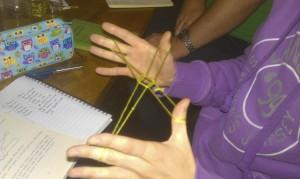… which then became a male turtle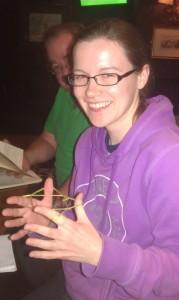And then Sarah graduated to string figure master with this very complicated pattern: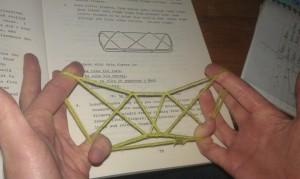### A couple of coin puzzles

I have $$N$$ coins. I place each of them in turn in piles. It’s equally likely that I’ll place a coin on any of the existing piles, or start a new pile. How many piles should I expect there to be when I’m done?

We didn’t come up with an answer. David, Stephen and Ji set to work computing numbers for small values of $$N$$. David demanded that I take a photo of his “maths explosion”.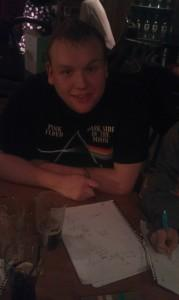Stephen and Ji were concentrating too hard to notice me take this picture.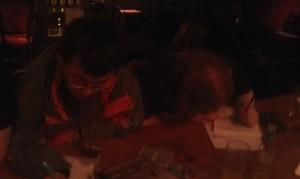I have a biased coin, but I don’t know what the bias is. How can I use it to make a fair decision between two choices?

If you want to think about this before reading the solution, here’s your warning that the next sentence will spoil it for you. The solution is to flip the coin twice until you get two different results, and take the result of the first flip as your answer. It works because, if the probability of getting heads is $$p$$, the probability of getting heads-then-tails and of getting tails-then-heads are the same: $$p \cdot (1-p)$$.

### Hanging pictures just about safely enough

When David got hold of the wool we’d been using to make the string figures of the Tuamotus, he asked us this:

How do you hang a picture from $$N$$ nails with a single piece of string, so that removing any one of the nails makes the picture fall?

David showed us the solutions for two and three nails (or fingers of a willing participant in our case), which involve wrapping the string in a particular pattern around the nails. I first saw this trick at a Maths Busking event, but I can never remember the solution. On googling, it turns out the Demaine brothers and others have written a big paper on the subject.

Somehow, my pair of scissors ended up with some string tied very tightly to one of the handles. Since we had no other cutting implements, we agreed that David would have to run relativistically quickly near a black hole with the scissors to cut the string off.

In preparation for my PGF talk the following week, I went through the zero-knowledge sudoku protocol with John.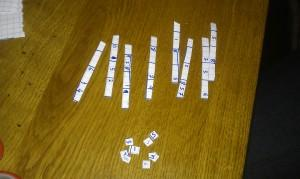Finally, we learned that googlewhacks don’t exist any more, because somebody has created two pages each containing every pair of words. I haven’t checked that fact; I hope it isn’t true.

The next Newcastle MathsJam is on the 20th of November, two days after the big MathsJam conference at Wychwood Park. I’m looking forward to both!

$1, \frac{3}{2}, \frac{23}{12}, \frac{41}{18}, \dotsc$
$-\frac{2}{3} + \sqrt{2\left(n + \frac{1}{3}\right)}$
The approximation gets better as $n$ increases and it looks like the error is $O(n^{-1.5})$.